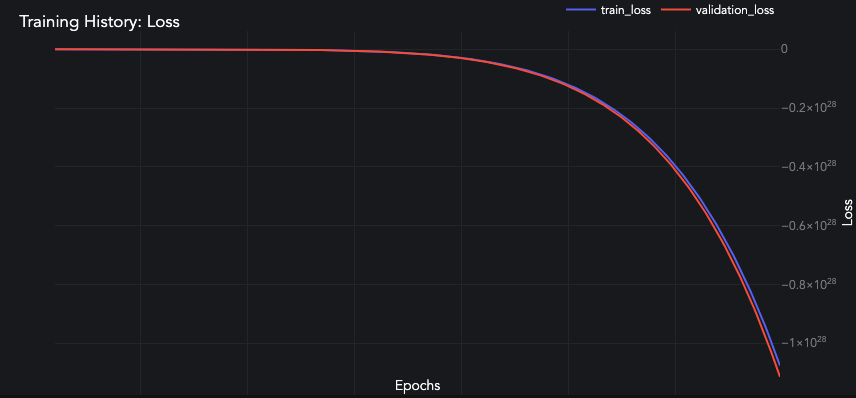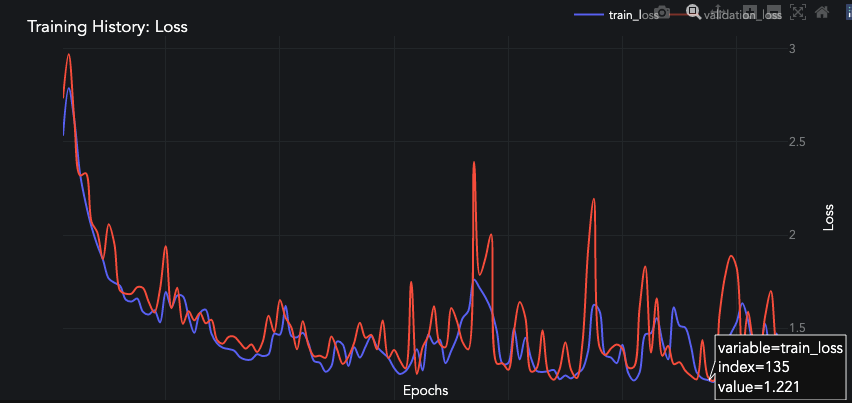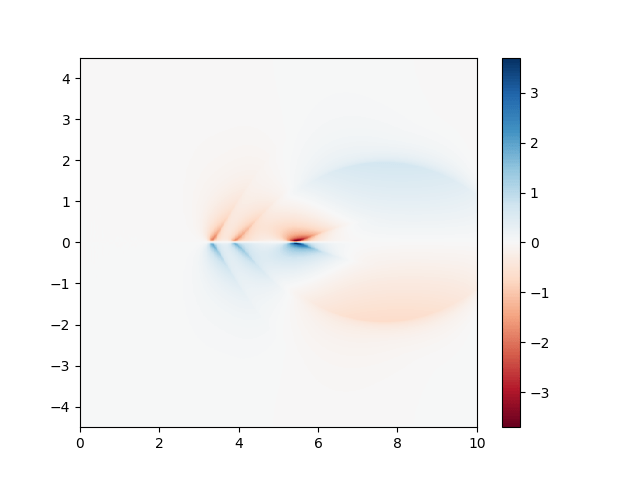## #StackBounty: #deep-learning #neural-network #convolutional-neural-network #autoencoder Autoencoder not learning walk forward image tra…

### Bounty: 50

I have a series of 15 frames with (60 rows x 50 columns). Over the course of those 15 frames, the moon moves from the top left to the bottom right.As my input data I have a 60×50 image. As my evaluation label I have a 60×50 image from 2 frames later. All are divided by 255.

I am attempting an autoencoder.

``````    model = keras.models.Sequential()

# last layer tried sigmoid with BCE loss.
# last layer tried relu with MAE.
``````

Tutorials say to use a final layer of sigmoid and BCE loss, but the values I’m producing must not be between 0-1 because the loss goes way negative.If I use a final layer of relu with MAE loss it claims to learn something.But the predicted image is notttt great:Get this bounty!!!

## #StackBounty: #python #neural-network #convolutional-neural-network #overfitting Is it possible to use a Neural Network to interpolate …

### Bounty: 50

I am completely new to Artificial intelligence and Neural Networks. I am currently working on a plasma physics simulation project which requires a very high resolution data set. We currently have the results of two simulations of the same problem run at different resolutions – with one’s resolution being higher than the other. However, we need an even higher resolution for us to use this data effectively. Unfortunately, it is not possible for us to run a higher resolution simulation because of computation power limitations. So instead, we are trying to somehow interpolate the data we have to get a reasonable estimate of what the simulation result might be if we were to run it at a higher resolution. I tried to interpolate the data using conventional interpolation techniques and functions in SciPy. However, the interpolated result is sometimes off by about 20 to 30 percent at certain points.

Problem Statement and my Idea

So I was wondering if it was possible to use a neural network to generate an output that when fed into the interpolator (code that I have written using SciPy), would yield better results than if I used just the interpolator. Currently, out data when plotted looks like this:This is the data plotted at a certain time t. However, we have data similar to this for about 30 different times steps – so we have 30 different data sets that look similar to this but are slightly altered. And as I said before, we also have the high resolution and low resolution data sets for each of the 30 timesteps.

My idea for the ANN is as follows: The low resolution data (a 512 x 256 2-D array) can be fed into a the network to output a slightly modified 512 x 256 2-D array. We can then input this modified data set into our interpolator and see if it matches the high resolution data set (1024 x 512). The error function for the network would be a function of the difference of the high data set and the interpolated data set (maybe something like the sum of the squares of the difference of each element in the arrays). This can then be done for all 30 different data sets to minimise the difference in the high res and interpolated data sets.

If this works as planned, I would somehow use this trained ANN to the high resolution data set (1024 x 512) to feed it’s output into the interpolator.

Questions

Is it possible to create a neural network that can do this, and if yes, what type of networks do this?

Even if the neural network can be trained, how do we upgrade to work for the high res data set (1024 x 512) when it was initially trained with the low res data set (512 x 256)?

Is this a trustworthy method to predict simulation results? (All 30 data sets look almost exactly like the image above; including the high res results)

Get this bounty!!!

## #StackBounty: #neural-network #regression #decision-trees #bert #embeddings Combining heterogeneous numerical and text features

### Bounty: 50

We want to solve a regression problem of the form "given two objects $$x$$ and $$y$$, predict their score (think about it as a similarity) $$w(x,y)$$". We have 2 types of features:

• For each object, we have about 1000 numerical features, mainly of the following types: 1) "Historical score info", e.g. historical means $$w(x,cdot)$$ up to the point we use the feature; 2) 0/1 features meaning whether object $$x$$ has a particular attribute, etc.
• For each object, we have a text which describes the object (description is not reliable, but still useful).

Clearly, when predicting a score for a pair $$(x,y)$$, we can use features for both $$x$$ and $$y$$.

We are currently using the following setup (I omit validation/testing):

• For texts, we compute their BERT embeddings and then produce a feature based on the similarity between the embedding vectors (e.g. cosine similarity between them).
• We split the dataset into fine-tuning and training datasets. The fine-tuning dataset may be empty meaning no fine-tuning.
• Using the fine-tuning dataset, we fine-tune BERT embeddings.
• Using the training dataset, we train decision trees to predict the scores.

We compare the following approaches:

• Without BERT features.
• Using BERT features, but without fine-tuning. There is some reasonable improvement in prediction accuracy.
• Using BERT features, with fine-tuning. The improvement is very small (but the prediction using only BERT features improved, of course).

Question: Is there something simple I’m missing in this approach? E.g. maybe there are better ways to use texts? Other ways to use embeddings? Better approaches compared with decision trees?

I tried to do multiple things, without any success. The approaches which I expected to provide improvements are the following:

• Fine-tune embeddings to predict difference between $$w(x,y)$$ and mean $$w(x, cdot)$$. The motivation is that we already have a feature "mean $$w(x,cdot)$$", which is a baseline for an object $$x$$, and we are interested in the deviation from this mean.

• Use NN instead of decision trees. Namely, I use few dense layers to turn embedding vectors into features, like this:

`````` nn.Sequential(
nn.Linear(768 * 2, 1000),
nn.BatchNorm1d(1000),
nn.ReLU(),
nn.Linear(1000, 500),
nn.BatchNorm1d(500),
nn.ReLU(),
nn.Linear(500, 100),
nn.BatchNorm1d(100),
nn.ReLU(),
nn.Linear(100, 10),
nn.BatchNorm1d(10),
nn.ReLU(),
)
``````

After that, I combine these new $$10$$ features with $$2000$$ features I already have, and use similar architecture on top of them:

``````  nn.Sequential(
nn.Linear(10 + n_features, 1000),
nn.BatchNorm1d(1000),
nn.ReLU(),
nn.Linear(1000, 500),
nn.BatchNorm1d(500),
nn.ReLU(),
nn.Linear(500, 100),
nn.BatchNorm1d(100),
nn.ReLU(),
nn.Linear(100, 1),
)
``````

But as a result, my prediction is much worse compared with decision trees. Are there better architectures suited for my case?

Get this bounty!!!

## #StackBounty: #python #tensorflow #machine-learning #neural-network #tf.keras TensorFlow/Keras Using specific class recall as metric fo…

### Bounty: 100

*Update at bottom

I am trying to use recall on 2 of 3 classes as a metric, so class B and C from classes A,B,C.

(The original nature of this is that my model is highly imbalanced in the classes [~90% is class A], such that when I use accuracy I get results of ~90% for prediciting class A everytime)

``````model.compile(
loss='sparse_categorical_crossentropy', #or categorical_crossentropy
optimizer=opt,
metrics=[tf.keras.metrics.Recall(class_id=1, name='recall_1'),tf.keras.metrics.Recall(class_id=2, name='recall_2')]
)

history = model.fit(train_x, train_y, batch_size=BATCH, epochs=EPOCHS, validation_data=(validation_x, validation_y), callbacks=[tensorboard, checkpoint])
``````

This spits out an error:

``````raise ValueError("Shapes %s and %s are incompatible" % (self, other))

ValueError: Shapes (None, 3) and (None, 1) are incompatible
``````

Model summary is:

``````Model: "sequential"
_________________________________________________________________
Layer (type)                 Output Shape              Param #
=================================================================
lstm (LSTM)                  (None, 120, 32)           19328
_________________________________________________________________
dropout (Dropout)            (None, 120, 32)           0
_________________________________________________________________
batch_normalization (BatchNo (None, 120, 32)           128
_________________________________________________________________
lstm_1 (LSTM)                (None, 120, 32)           8320
_________________________________________________________________
dropout_1 (Dropout)          (None, 120, 32)           0
_________________________________________________________________
batch_normalization_1 (Batch (None, 120, 32)           128
_________________________________________________________________
lstm_2 (LSTM)                (None, 32)                8320
_________________________________________________________________
dropout_2 (Dropout)          (None, 32)                0
_________________________________________________________________
batch_normalization_2 (Batch (None, 32)                128
_________________________________________________________________
dense (Dense)                (None, 32)                1056
_________________________________________________________________
dropout_3 (Dropout)          (None, 32)                0
_________________________________________________________________
dense_1 (Dense)              (None, 3)                 99
=================================================================
Total params: 37,507
Trainable params: 37,315
Non-trainable params: 192
``````

Note that the model works fine without the errors if using:

``````metrics=['accuracy']
``````

but this and this made me think something has not been implemented along the lines of tf.metrics.SparseCategoricalRecall()

from

tf.metrics.SparseCategoricalAccuracy()

So I diverted to a custom metric which decended into a rabbit hole of other issues as I am highly illeterate when it comes to classes and decorators.

I botched this together from an custom metric example (I have no idea how to use the sample_weight so I commented it out to come back to later):

``````class RelevantRecall(tf.keras.metrics.Metric):

def __init__(self, name="Relevant_Recall", **kwargs):
super(RelevantRecall, self).__init__(name=name, **kwargs)

def update_state(self, y_true, y_pred, sample_weight=None):
y_pred = tf.argmax(y_pred, axis=1)
report_dictionary = classification_report(y_true, y_pred, output_dict = True)

# if sample_weight is not None:
#     sample_weight = tf.cast(sample_weight, "float32")
#     values = tf.multiply(values, sample_weight)

def result(self):
return self.joined_recall

def reset_states(self):
# The state of the metric will be reset at the start of each epoch.
self.joined_recall.assign(0.0)

model.compile(
loss='sparse_categorical_crossentropy', #or categorical_crossentropy
optimizer=opt,
metrics=[RelevantRecall()]
)

history = model.fit(train_x, train_y, batch_size=BATCH, epochs=EPOCHS, validation_data=(validation_x, validation_y), callbacks=[tensorboard, checkpoint])
``````

This aim is to return a metric of `[recall(b)+recall(c)/2]`. I’d imagine returning both recalls seperately like `metrics=[recall(b),recall(c)]` would be better but I can’t get the former to work anyway.

I got a tensor bool error: `OperatorNotAllowedInGraphError: using a 'tf.Tensor' as a Python 'bool' is not allowed: AutoGraph did convert this function. This might indicate you are trying to use an unsupported feature.` which googling led me to add: `@tf.function` above my custom metric class.

This led to a old vs new class type error:

``````super(RelevantRecall, self).__init__(name=name, **kwargs)
TypeError: super() argument 1 must be type, not Function
``````

which I didn’t see how I had achieved since the class has an object?

As I said I’m quite new to all aspects of this so any help on how to achieve (and how best to achieve) using a metric of only a selection of prediciton classes would be really appreciated.

OR

Ideally I’d like to go with the former method of using `tf.keras.metrics.Recall(class_id=1....` as it seems the neatest way if it worked.

I am able to get the recall for each class when using a similar function in the callbacks part of the model, but this seems more intensive as I have to do a model.predict on val/test data at the end of each epoch.
Also unclear if this even tells the model to focus on improving the selected class (i.e difference in implementing it in metric vs callback)

Callback code:

``````class MetricsCallback(Callback):
def __init__(self, test_data, y_true):
# Should be the label encoding of your classes
self.y_true = y_true
self.test_data = test_data

def on_epoch_end(self, epoch, logs=None):
# Here we get the probabilities - longer process
y_pred = self.model.predict(self.test_data)

# Here we get the actual classes
y_pred = tf.argmax(y_pred,axis=1)
report_dictionary = classification_report(self.y_true, y_pred, output_dict = True)
print ("n")

print (f"Accuracy: {report_dictionary['accuracy']} - Holds: {report_dictionary['0.0']['recall']} - Sells: {report_dictionary['1.0']['recall']} - Buys: {report_dictionary['2.0']['recall']}")
self._data = (float(report_dictionary['1.0']['recall'])+float(report_dictionary['2.0']['recall']))/2
return

metrics_callback = MetricsCallback(test_data = validation_x, y_true = validation_y)

history = model.fit(train_x, train_y, batch_size=BATCH, epochs=EPOCHS, validation_data=(validation_x, validation_y), callbacks=[tensorboard, checkpoint, metrics_callback)
``````

# Update 19/07/2021

• I have resorted to using `categorical_crossentropy` for `loss` instead of `sparse_categorical_crossentropy`.
• One-hot-encoding my class/target arrays.
• Using tf recall: `[tf.keras.metrics.Recall(class_id=1, name='recall_1')`

I am now using the code below.

``````train_y = tf.one_hot(train_y, 3)
validation_y = tf.one_hot(validation_y, 3)
test_y = tf.one_hot(test_y, 3)

model.compile(
loss='categorical_crossentropy',
optimizer=opt,
metrics=[tf.keras.metrics.Recall(class_id=1, name='No'),tf.keras.metrics.Recall(class_id=2, name='Yes')]
) #tf.keras.metrics.Recall(class_id=0, name='Wait')

history = model.fit(train_x, train_y, batch_size=BATCH, epochs=EPOCHS, validation_data=(validation_x, validation_y), callbacks=[tensorboard, checkpoint])
``````

Thanks to Abhishek Prajapat

This achieves the same overall goal and probably has a very small difference/impact on performance due to a small number of mutually exclusive classes,

but in the case of a very large number of mutually exclusive classes I still don’t have an solution to achieving the same goal as above using `sparse_categorical_crossentropy`

Get this bounty!!!

## #StackBounty: #tensorflow #machine-learning #neural-network #lstm #recurrent-neural-network Keras LSTM input ValueError: Shapes are inc…

### Bounty: 50

Not sure about why I’m getting an error with my LSTM neural network. It seems to be related with the input shape.

This is my neural network architecture:

``````from keras.models import Sequential
from keras.layers import LSTM, Dense, Dropout

model = Sequential()

# Recurrent layer
dropout=0.1, recurrent_dropout=0.1))

# Fully connected layer

# Dropout for regularization

# Output layer

# Compile the model
``````

This is how I train it:

``````history = model.fit(X_train_padded, y_train_padded,
batch_size=2048, epochs=150,
``````

This is the shape of my input data:

``````print(X_train_padded.shape, X_test_padded.shape, y_train_padded.shape, y_test_padded.shape)
(98, 20196, 30) (98, 4935, 30) (98, 20196, 1) (98, 4935, 1)
``````

This is part of my X_train_padded:

``````X_train_padded
array([[[ 2.60352379e-01, -1.66420518e-01, -3.12893162e-01, ...,
-1.51210476e-01, -3.56188897e-01, -1.02761131e-01],
[ 1.26103191e+00, -1.66989382e-01, -3.13025807e-01, ...,
6.61329839e+00, -3.56188897e-01, -1.02761131e-01],
[ 1.04418243e+00, -1.66840157e-01, -3.12994596e-01, ...,
-1.51210476e-01, -3.56188897e-01, -1.02761131e-01],
...,
[ 1.27399408e+00, -1.66998426e-01, -3.13025807e-01, ...,
6.61329839e+00, -3.56188897e-01, -1.02761131e-01],
``````

This is the error that I’m getting:

``````Epoch 1/150
---------------------------------------------------------------------------
ValueError                                Traceback (most recent call last)
<ipython-input-52-52422b54faa4> in <module>
2                     batch_size=2048, epochs=150,
...
ValueError: Shapes (None, 20196) and (None, 12) are incompatible
``````

As I’m using a LSTM layer, I have a 3D input shape. My output layer has 12 nodes (y_train.nunique()) because I have 12 different classes in my input. Given that I have 12 classes, I’m using softmax as activation function in my output layer and categorical_crossentropy as my loss function.

Get this bounty!!!

## #StackBounty: #python #tensorflow #keras #neural-network #recurrent-neural-network Setting the initial state of an RNN represented as a…

### Bounty: 50

How do I set the initial state of the recurrent neural network `rnn` constructed below?

``````from tensorflow.keras.layers import Dense, SimpleRNN
from tensorflow.keras.models import Sequential

rnn = Sequential([SimpleRNN(3), Dense(1)])
``````

I’d like to specify the initial state of the first layer before fitting the model with `model.fit`.

Get this bounty!!!

## #StackBounty: #deep-learning #neural-network #convolutional-neural-network Non Linearity used in LeNet 5

### Bounty: 50

I was looking at the original implementation of LeNet-5 and I noticed a disparity in different sources. Wikipedia suggests that the non linearity used is the same sigmoid in each layer, some blog posts use a combination of Tanh and sigmoid while Andrew NG said it used some crude non linearity which no one uses today without naming it. I looked at the original paper but it’s like 50 pages long and the diagram does not mention the activation functions used explicitly. I searched a bit and the sigmoid function was there and mentioned in context of activations while the tanh function is taken as a squashing function. I’m not sure if that is the same or different as then it used other terms when referring to the sigmoid ones. Anyone knows what’s up with this?

Get this bounty!!!

## #StackBounty: #machine-learning #deep-learning #neural-network #classification #feature-extraction Deciding which samples the model wil…

### Bounty: 100

Problem:

Given a neural network for image classification, the objective is to develop an algorithm which decides which images are ‘problematic’ and the model is probably going to classify them incorrectly.

Discussion:

So far, I’ve thought of two possible approaches:

• Feed the given image to the model and then analyse its softmax ouput with various metrics (difference between first and second class confidence, entropy, gini index etc).
• Perform some kind of image processing (feature extraction) on the given image, to obtain some features that indicate whether the image is not going to be correctly classified.

Questions:

Can you provide me with more suggestions about the second approach? What type of feature extraction would you think will help distinguish those images?

Any other ideas that are not mentioned here are welcome.

Get this bounty!!!

## #StackBounty: #machine-learning #neural-network #deep-learning Why is the "dying ReLU" problem not present in most modern dee…

### Bounty: 50

The $$ReLU(x) = max(0,x)$$ function is an often used activation function in neural networks.
However it has been shown that it can suffer from the dying Relu problem (see
also What is the "dying ReLU" problem in neural networks?)

Given this problem with the ReLU function and the often seen suggestion to use a leaky ReLU instead, why is it that to this day ReLU remains the most used activation function in modern deep learning architectures? Is it simply a theoretical problem that does not often occur in practice? And if so, why does it not occur often in practice? Is it because as the width of a network becomes larger the probability of dead ReLUs becomes smaller (see Dying ReLU and Initialization: Theory and Numerical Examples
)?

We moved away from sigmoid and tanh activation functions due to the vanishing gradient problem and avoid RNN’s due to exploding gradients but it seems like we haven’t moved away from ReLUs and their dead gradient? I would like to get more insights in to why.

Get this bounty!!!

## #StackBounty: #matlab #deep-learning #neural-network #time-series #conv-neural-network What is the purpose of a sequence folding layer …

### Bounty: 50

When designing a CNN for 1D time series signal classification in MATLAB i get the error that the 2dconvolutional layer does not take sequences as input. From my understanding it is perfectly possible to convolve of an "array" with a 3×1 filter. To resolve this issue MATLAB suggests to use a "sequence folding layer". What would be the function of such a sequence folding layer and how would the architecture need to be changed?

I get the following error message:Get this bounty!!!